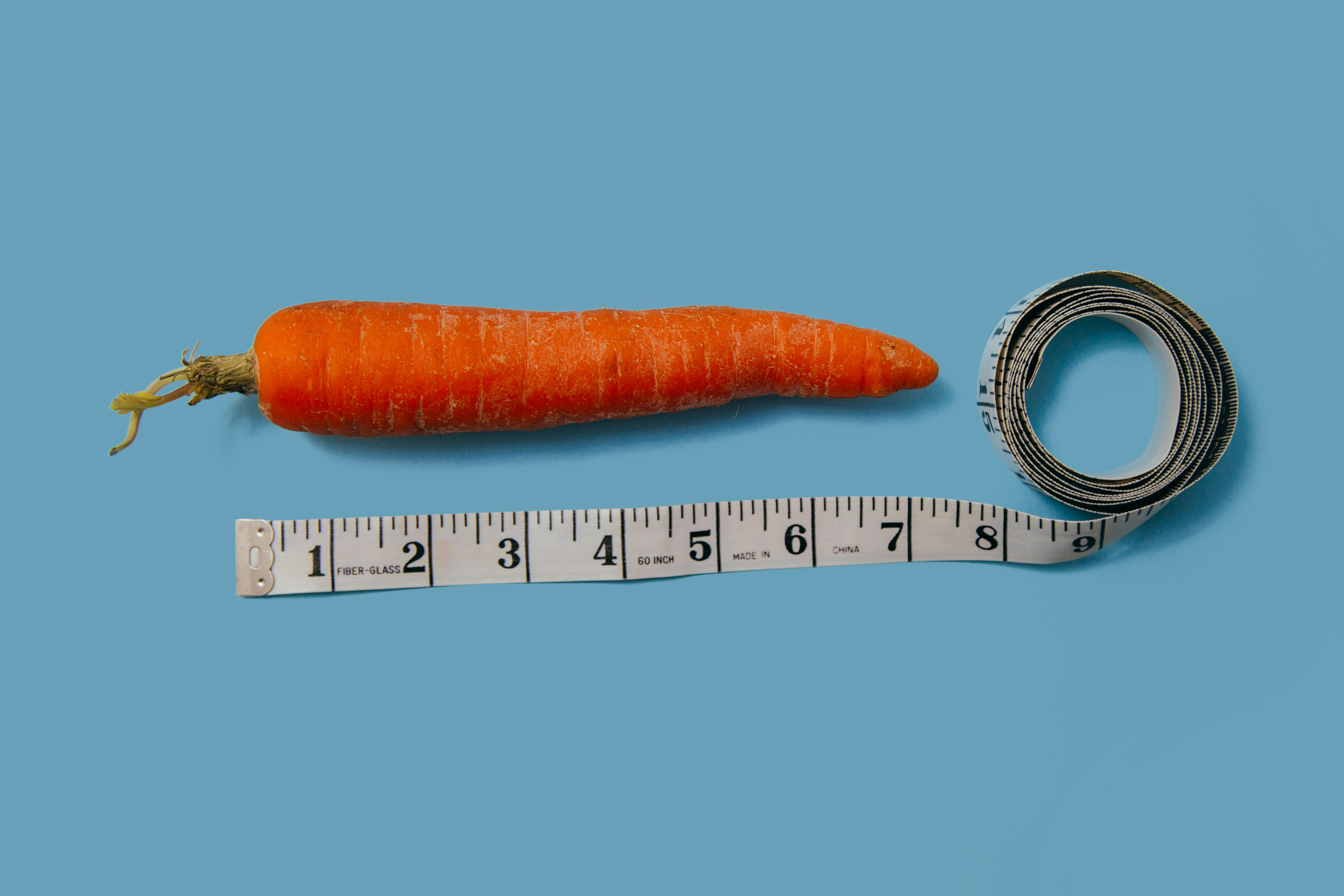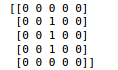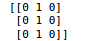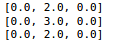arsalanwasim6@gmail.com

# Average PoolingConvolution layers are great in recording the exact area of highlights of the picture. A major issue for convolution neural networks is that they compute the particular highlights from a given picture and any adjustment of the picture, for example, zooming, panning and some other actual change brings about mistaken forecasts at the end of the day you can say that they are interpretation variation implies they will be changed if there is any actual interpretation.

To make the learned features more smooth average pooling is commonly used which results in downsampling the input image by learning the average features in (2,2) window located in the result of the previous convolution. Let us clarify this with an example.

Suppose that we have an input two-dimensional padded matrix:We will use a vertical line detector kernel to detect vertical lines; the filter is also a two-dimensional matrix and would look as follows:The result of convolution operation would look as:Now we will use the average-pooling layer to learn the average features in a (2,2) window from the resultant matrix, the operation looks as follows:

Average (0.0, 2.0)           =     [1.25]

(0.0, 3.0)

Since our output from the convolution operation is a (3,3) matrix, average-pooling would only look at the first (2,2) window and by default average pool layer has a stride of 2 so it will skip the others as it will be out of its scope but would output the most average feature calculated as:

Average = (0.0 + 2.0+ 0.0+ 3.0)/4 = [1.25]

Average pooling makes the convolution operation smoother. It adds a small amount of translation invariance, which means that if input images are translated by a small amount, the result will not be affected.